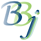# BBjNumber::intValue

## Description

In BBj 7.0 and higher, this method returns the value of the specified number as an int, which may involve rounding or truncation.

Return Value

Method

int

intValue()

None.

## Return Value

Returns the int value represented by this number.

None.

## Example

 ``````rem ' BBjNumber print "BBjNumber sample code" n! = 3.14159 print "n!=",n!.toString() print "n!.booleanValue()=",n!.booleanValue() print "n!.byteValue()=",n!.byteValue() print "n!.doubleValue()=",n!.doubleValue() print "n!.equals(3.14159))=",n!.equals(3.14159) print "n!.floatValue()=",n!.floatValue() print "n!.hashCode()=",n!.hashCode() print "n!.intValue()=",n!.intValue() print "n!.longValue()=",n!.longValue() print "n!.shortValue()=",n!.shortValue()``````